##Pascal's theorem

The interesection points of the opposite sides of a hexagon inscribed in a circle (more generally in a conic) are collinear (on a line named a Pascal line of the hexagon (red line in the figure)).
The term opposite sides needs an explanation. Given a contiguous ordering ABCDEF of the vertices of the hexagon, opposite sides are defined by taking two successive vertices f.e. CD, leaving one and taking the next two (modulo cyclic permutation of the letters) i.e. FA defining point O in this example.
Pascal's theorem is valid more generally for conics, even for degenerate such. F.e. two intersecting (or parallel) lines can be considered to form a degenerate conic. In this case Pascal's theorem is identical with the theorem of Pappus on hexagons inscribed in two lines (see PappusLines.html ).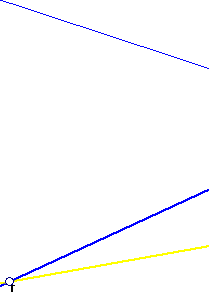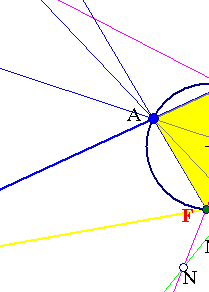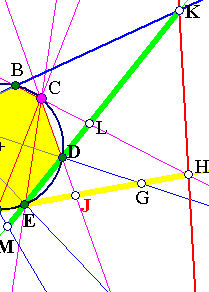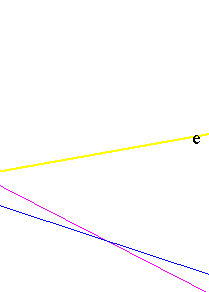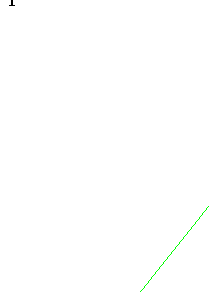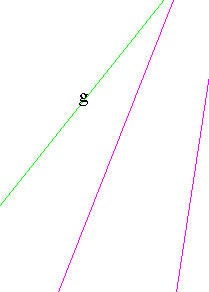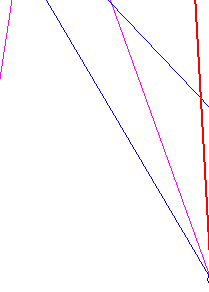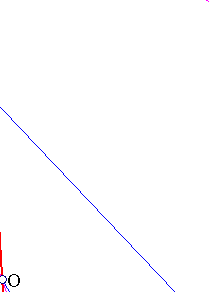At first glance the theorem seems to be formidable and need a complicated proof argument. It is though a simple consequence of the properties of cross ratios (see CrossRatio0.html ).
The cross ratios (FEJH) and (MEDK) are equal, hence lines FM, DJ and KH are concurrent. This simple proof may be transferred verbatim to the more general case of an hexagon inscribed in a conic. All the ingredients of the proof are valid in this more general setting.
The cross ratios (FEJH) and (MEDK) are equal because they are cut off on lines e, g by the line bundles C(FEDB) and A(FEDB). But the four points (FEDB) as well as A, C being on a circle(conic) the cross ratios of these line bundles are equal (see CrossRatio.html ).
Pascal's theorem has several consequences and particular cases, examined in the references given below. It has also an obvious converse (called Maclaurin-Braikenridge theorem).
Converse: If the opposite sides of a hexagon intersect at three collinear points then the hexagon is inscribed in a conic (may be degenerate). Consider for example in the figure above the conic (circle) passing through the five (out of the six) points A,...,E. If that conic passes through a point F' of line EH, then line EF' (after Pascal) should intersect CD at O. If follows that F' is on line AO hence coincides with F.

Brianchon.html
CrossRatio.html
CrossRatio0.html
CrossRatioLines.html
Duality.html
GoodParametrization.html
Harmonic.html
Harmonic_Bundle.html
HomographicRelation.html
HomographicRelationExample.html
PappusLines.html
Pascal2.html
PascalImmortel.html